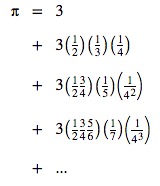# A Million Digits of Pi in 9 Lines of Javascript

"Big integers" have landed in Javascript, at least in Firefox and Chrome. One of my favorite things to do with high precision arithmetic is to calculate digits of π. From scratch, that is, using only addition, subtraction, multiplication, and division.

You can find lots of formulas for calculating π, but my favorite is this one:For some reason, it doesn't make most lists of π formulas, but I like it because of its simplicity. I can recall it easily without looking anything up. All you have to remember are the first two terms and a simple evolution rule for each of the three multiplicands. An uncle told it to me when I was in elementary school and I've been able to remember it for decades. I never even knew why it worked until I investigated it this month. (Hint: Taylor series)

It only uses addition, multiplication, and division. No square roots. It computes π directly (not 1/π). And even though there are complicated formulas that converge faster, this simple one still converges at about 0.6 decimal digits per term.

To use big integers in Javascript, you put an "n" suffix on your integer literals. Here is how we can use this formula to calculate the first thousand digits of π in Javascript:

```let i = 1n;
let x = 3n * (10n ** 1020n);
let pi = x;
while (x > 0) {
x = x * i / ((i + 1n) * 4n);
pi += x / (i + 2n);
i += 2n;
}
console.log(pi / (10n ** 20n));
```

We just calculate the terms of the sequence with the decimal point shifted right 1020 places (using BigInts), until they are too small to matter, add them up, chop off the last 20 digits, and print the result.

This code works as is in Chrome, Firefox, and the latest version of Nodejs. Here are execution times on a 2014 MacBook Pro (4-core i7 2.50 GHz, though I'm sure it's only using one core.)

Length Firefox 68 Chrome 76 Node 10
1000 digits  0.02 sec  0.01 sec  0.01 sec
10,000 digits  1.04 sec  0.34 sec  0.40 sec
100,000 digits 88.91 sec 30.74 sec 37.34 sec

Increasing the number of digits computed is as simple as increasing the exponent in line 2, so here is Javascript code to generate a million digits of π:

```let i = 1n;
let x = 3n * (10n ** 1000020n);
let pi = x;
while (x > 0) {
x = x * i / ((i + 1n) * 4n);
pi += x / (i + 2n);
i += 2n;
}
console.log(pi / (10n ** 20n));
```

This works in the Chrome developer console. It takes about an hour on my machine. Firefox, however, seems to hang when dealing with numbers longer than 315,633 digits (which happens to be right around 1,048,576 bits).

Here is a page that computes π in your browser. It uses the code above, with some modifications so it can show progress as it goes.